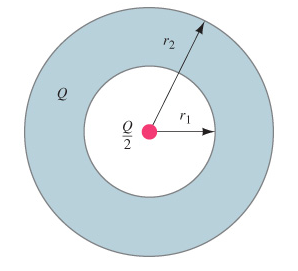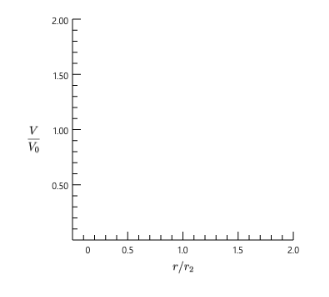# Problem: A hollow spherical conductor, carrying a net charge +Q, has inner radius r1 and outer radius r2=2r1 (see the figure (Figure 1)). At the center of the sphere is a point charge +Q/2. Plot V as a function of r from r=0 to r=2r2. Assume V0=3Q/8πϵ0r2.

###### FREE Expert Solution

Since r > r2:

$\mathbit{E}\mathbf{=}\frac{\mathbf{\left(}\mathbf{3}\mathbf{Q}}{\mathbf{2}}\mathbf{\right)}}{\mathbf{4}\mathbf{\pi }{\mathbf{\epsilon }}_{\mathbf{0}}{\mathbf{r}}^{\mathbf{2}}}$

We have:

$\begin{array}{rcl}\mathbf{V}& \mathbf{=}& \mathbf{\int }\frac{\mathbf{3}\mathbf{Q}}{\mathbf{8}\mathbf{\pi }{\mathbf{\epsilon }}_{\mathbf{0}}{\mathbf{r}}^{\mathbf{2}}}\mathbf{·}\mathbf{d}\mathbf{r}\\ & \mathbf{=}& \frac{\mathbf{3}\mathbf{Q}}{\mathbf{8}\mathbf{\pi }{\mathbf{\epsilon }}_{\mathbf{0}}\mathbf{r}}\end{array}$

91% (439 ratings)###### Problem Details

A hollow spherical conductor, carrying a net charge +Q, has inner radius rand outer radius r2=2r1 (see the figure (Figure 1)). At the center of the sphere is a point charge +Q/2. Plot V as a function of r from r=0 to r=2r2. Assume V0=3Q/8πϵ0r2.Frequently Asked Questions

What scientific concept do you need to know in order to solve this problem?

Our tutors have indicated that to solve this problem you will need to apply the Electric Potential concept. You can view video lessons to learn Electric Potential. Or if you need more Electric Potential practice, you can also practice Electric Potential practice problems.

What professor is this problem relevant for?

Based on our data, we think this problem is relevant for Professor Smecker Hane's class at UCI.